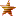###Author Topic: The mc-Dev Board Introduction  (Read 157 times)

#### mcThings

• We Connect Things To The Internet... That Can't Be Plugged In.
• Jr. Member
••• Posts: 68##### The mc-Dev Board Introduction
« on: July 19, 2016, 11:18:10 am »
The mc-Dev Board Introduction
In this video we have Tom and Josh introducing the re-designed mc-Dev board. Josh will go over functions and values of this product. The guys will then showcase a use-case.

Follow below to gain the code for the use-case shown in the video.

The code will also be posted in our forum that can be accessed through our website.

Cheers
- The mc-Team

https://youtu.be/b6JoMtgul4M

Code: [Select]
`Define PinMode Pin2 As AnalogInput Alias lightVoltage Define PinMode Pin3 As DigitalOutput Define PinMode Pin4 As DigitalOutput Define PinMode Pin5 As DigitalOutput Define PinMode Pin6 As DigitalOutput Define PinMode Pin7 As DigitalOutput Define PinMode Pin8 As DigitalOutput Class ldrTest Shared Event Boot() Pin3 = False Pin4 = False Pin5 = False Pin6 = False Pin7 = False Pin8 = False LedRed = False LedGreen = False End Event Shared Event measureLight() RaiseEvent Every 50 milliSeconds Thread.Sleep(20000) 'sleep 20ms for voltage to stabilize Dim voltage As Short = lightVoltage If voltage > 3000 Then Pin3 = True Pin4 = True Pin5 = True Pin6 = True Pin7 = True Pin8 = True ElseIf voltage <= 3000 And voltage >= 2500 Then Pin3 = False Pin4 = True Pin5 = True Pin6 = True Pin7 = True Pin8 = True ElseIf voltage <= 2499 And voltage >= 2000 Then Pin3 = False Pin4 = False Pin5 = True Pin6 = True Pin7 = True Pin8 = True ElseIf voltage <= 1999 And voltage >= 1500 Then Pin3 = False Pin4 = False Pin5 = False Pin6 = True Pin7 = True Pin8 = True ElseIf voltage <= 1499 And voltage >= 1000 Then Pin3 = False Pin4 = False Pin5 = False Pin6 = False Pin7 = True Pin8 = True ElseIf voltage < 999 And voltage >= 500 Then Pin3 = False Pin4 = False Pin5 = False Pin6 = False Pin7 = False Pin8 = True ElseIf voltage < 500 Then Pin3 = False Pin4 = False Pin5 = False Pin6 = False Pin7 = False Pin8 = False Else End If End Event 'Battery check Shared Event measureVoltage() RaiseEvent Every 2 Hours Dim BattVolt As Short = Device.BatteryVoltage If BattVolt < 2200 Then Lplan.IFTTT("mjgu7QocyzTNDUbzgitzHxBYPFaXckJrJ2LbLq8XMMg", "GarageBatt") Else End If End Event End Class `